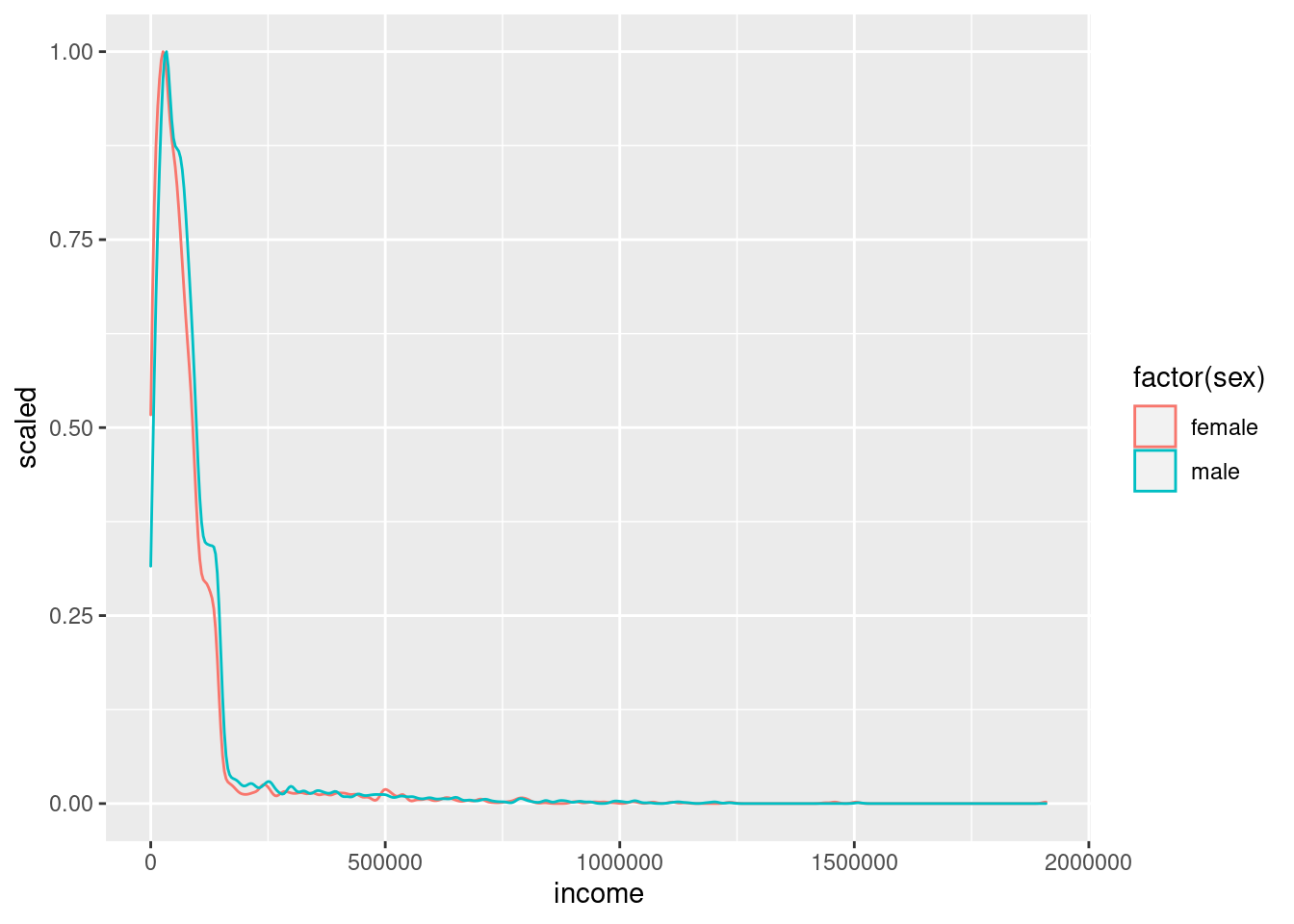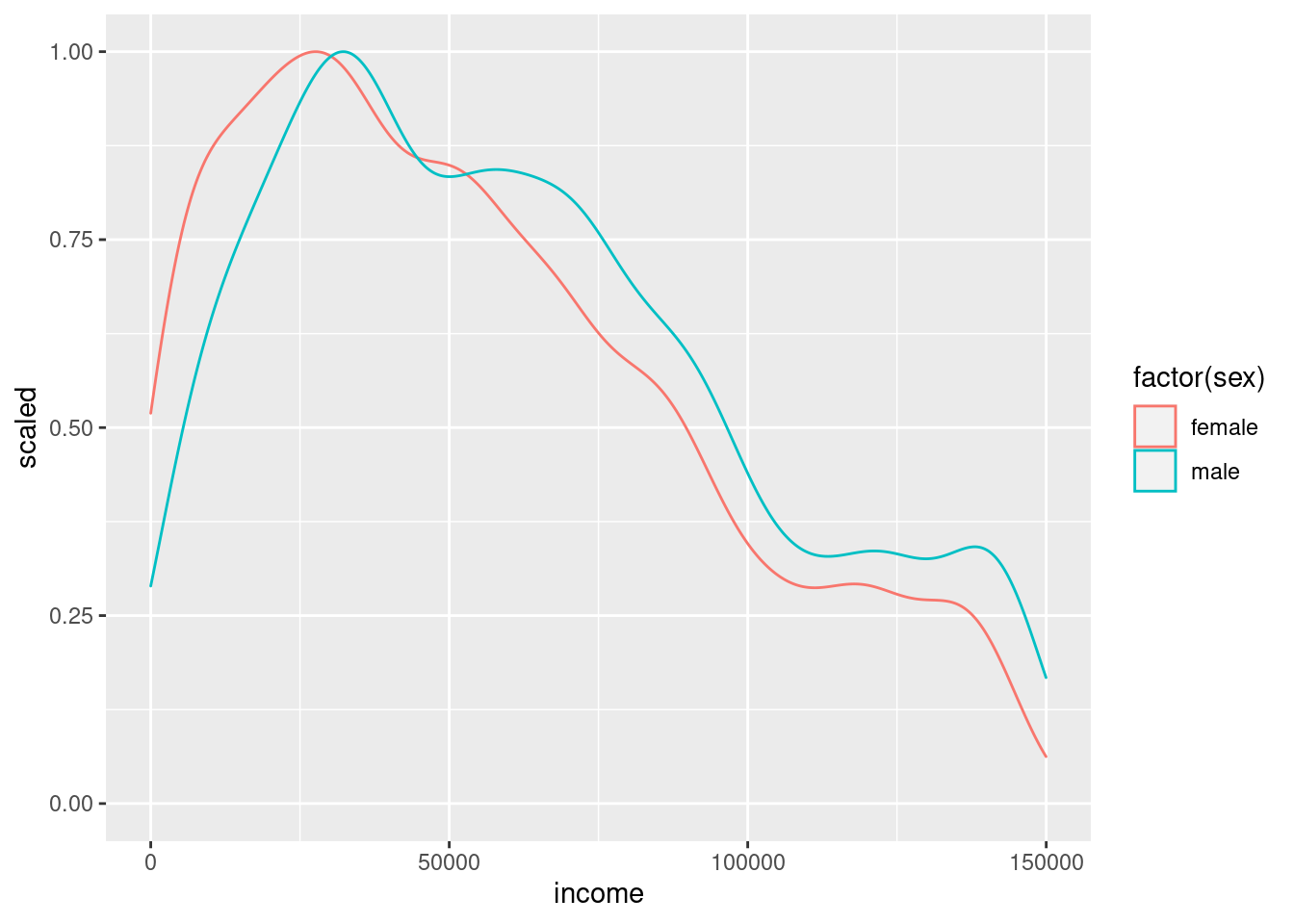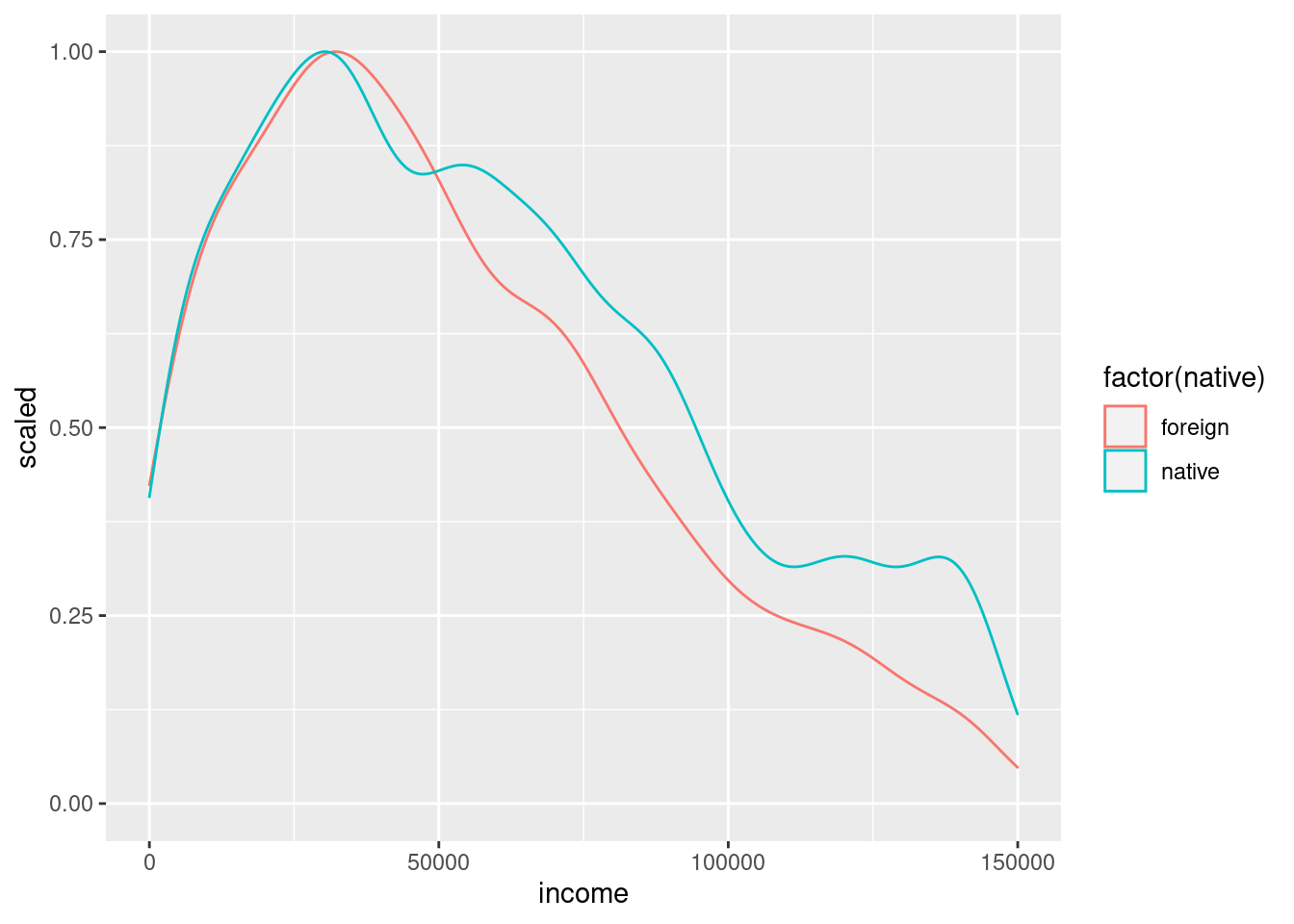## Before you start…

Before starting this worksheet, you should have had a brief introduction to using RStudio – Using RStudio. You should also have also completed the worksheet Exploring Data. If not, take a look these earlier worksheets before continuing.

If you have completed those worksheets, then you’ll have set up an R project, and you’ll have a script in it that looks like this:

# Exploring data (briefly)
library(tidyverse)
# Display mean income
cpsdata %>% summarise(mean(income))
# Calculate mean hours per week
cpsdata %>% summarise(mean(hours, na.rm = TRUE))

In this worksheet, we’ll add some more commands to this script.

## Grouping data

One of the most widely discussed issues concerning income is the difference between what men and women, on average, get paid. Let’s have a look at that difference in our teaching sample of 10,000 US participants.

In order to do this, we need to split our data into two groups – males and females. In R, the command group_by allows us to do this. In this case, we want to group the data by biological sex, so the command is group_by(sex). We pipe (%>%) the data in cpsdata to the group_by command in order to group it, and then we pipe (%>%) it to summarise to get a summary for each group (a mean, in this case). So, the full command is:

# Group differences (briefly)
# Group by sex, display mean income
cpsdata %>% group_by(sex) %>% summarise(mean(income))
# A tibble: 2 × 2
sex    mean(income)
<chr>           <dbl>
1 female         82677.
2 male           92137.

Copy it into your script and run it (CTRL+ENTER). The message concerning “ungrouping output” just means that after R gives you the means, it forgets that you grouped the data (so if you want to use these groups again, you’ll have to ask for them again).

Women in our made-up sample get paid, on average, around 9,000 (9k) less than men. Of course, not every male gets 92k a year in the US, and not every female gets 83k. It seems very likely that the range of incomes earned by men and women overlap – meaning that if you picked one man and one woman at random, there’s a reasonable chance that the woman earns more than the man. We can look at this variation in pay using a graph.

### Looking at variation using a density plot

The graph we’re going to draw is a density plot. If you recall histograms from school, it’s a lot like that. If not, don’t worry. A density plot is a curve that shows how likely a range of incomes are. So, the higher the curve is at a particular income, the more people who have that income.

We’re going to produce what’s called a scaled density plot. The highest point on a scaled density plot is always one. This can make it easier to compare two groups, particularly if one group has fewer people in it than the other.

So here’s the comment and command to do a scaled density plot for incomes, plotting men and women separately. Copy it into your script and run it (CTRL+ENTER).

# Display density plot of income, by sex
cpsdata %>% ggplot(aes(income, colour = factor(sex))) + geom_density(aes(y = ..scaled..))### Explanation of command

Here’s what each part of this command means:

cpsdata - The data frame containing the data. You created this in the last worksheet.

%>% - A pipe. As in the last worksheet, this pipe carries the data in cpsdata to the next part of the command, which does something with it.

ggplot() - This means ‘draw me a graph’. All graphs we use in these worksheets use the Grammar for Graphics (gg) plotting commands, so they’ll all include the command ggplot.

aes() - Short for aesthetics (what things look like). It means ‘This is the sort of graph I want’.

income - I want a graph of the data in the income column of cpsdata

color=factor(sex) - I want you to give me two graphs on top of each other, in different colours. One colour for men, a different color for women. Use the sex column of cpsdata to work out who is male and who is female.

geom_density() - I want this graph to be a density plot.

aes(y=..scaled..) - I want this density plot to be scaled (see above).

### Discussion of output

Your graph will appear in the bottom-right window, and should look like the one above. You’ll notice that the two lines seem basically on top of each other … but they can’t be because we know the two groups differ in mean income by over nine thousand dollars! We have a problem to solve…

## Dealing with extreme data points

The problem is one of scale – there are a small number of people who earn very high salaries. In fact, both the highest-paid man, and the highest-paid woman in our sample earn considerably more than one million dollars a year.

### Filtering data

Somehow, we need to deal with the fact that a few people in our sample are very well paid, which makes the difference between men and women hard to see on our graph, despite the difference being over nine thousand dollars a year. One of the easiest ways around this is to exclude these very high salaries from our graph.

The vast majority of people are paid less than 150k a year. So, let’s restrict our plotting to just those people. We do this using the filter command. It’s called filter because it works a bit like the filter paper in a chemistry lab (or in your coffee machine) – stopping some things, while letting other things pass through. We can filter our data by telling R what data we want to keep. Here, we want to keep all people who earn less than £150k, and filter out the rest. So the filter we need is filter(income < 150000), where < means “less than”.

We’ll be using this dataset of people with <$150k incomes a few times, so we’re going to give it a new name, cpslow (or any other name you want, e.g. angelface ) So, what we need to do is pipe (%>%) our cpsdata data to our filter(income < 150000), and use an arrow, <-, to send this data to our new data frame, cpslow. Recall that <- sends the thing on its right to the thing on its left, so the full command is: # Filter people with income <$150K into 'cpslow'
cpslow <- cpsdata %>% filter(income < 150000)

We can take a look at this new data frame by clicking on it in RStudio’s Environment window. By looking at the ID numbers, you can see that some people in our original sample have been taken out, because they earned at least 150k.

Now, we can plot these filtered data in the same way as before, by changing the name of the dataframe from cpsdata to cpslow.

So start with the command cpsdata %>% ggplot(aes(income, colour=factor(sex))) + geom_density(aes(y=..scaled..)), copy it onto the next line in your script, make that change, and press CTRL+RETURN. Also add a comment above your new command to explain what it does.

If you’ve got it right, your graph will look like this:At first glance, the two distributions of incomes still look quite similar. For example, the modal income – the point where the graph is highest – is at quite a low income, and that income is quite similar for both men and women. However, on closer inspection, you’ll also see that the red line (females) is above the blue line (men) until about 25-50k, and below the blue line from then on. This means that more women than men earn less than 50k, and more men than women earn more than 50k.

So, the gender pay gap is visible in this graph. The graph also illustrates that the difference in this sample is small, relative to the range of incomes. This doesn’t mean that the gender pay gap is less (or more) important than income inequality. These kinds of questions of importance are moral, philosophical, and political. Data cannot directly answer these kinds of questions, but they can provide information to inform the debate.

## Exercise

In this exercise, you’ll consolidate what you’ve learned so far.

The task is to further exmaine this sample of participants who are living in the US, and earning less than \$150k (cpslow).

Specifically, the question to answer is whether people born in the US earn more. In order to do this, you should calculate the mean income for each group, and produce a density plot with one line for each group. Below are the answers you are aiming for:

# A tibble: 2 × 2
native  mean(income)
<chr>            <dbl>
1 foreign         51423.
2 native          58905.Enter the following into your script:

# EXERCISE

, followed by the comments and commands you need to complete this exercise.

## Extension exercise

If you’ve some spare time and are looking for something a bit more challenging, try Exercise 2 on this slightly more advanced worksheet.